7 days of PowerPoint templates, graphics & videos - for free!* Unlimited asset downloads! Start 7-Day Free Trial

# How to Work With Excel Math Formulas (Guide to the Basics)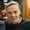Read Time: 8 mins

It's hard to get excited about learning math. It's something that most of us spend our lives avoiding. It's also one of the best reasons to use Microsoft Excel for perfect calculations, every time.

Don't think of these Excel formulas as math for math's sake. Instead, imagine how these formulas can help you automate your life and skip the trouble of making manual calculations. At the end of this tutorial, you'll have the skills you need to do all of the following, for example:

• Calculate the average score of your exams.
• Quickly subtotal the invoices you've issued to clients.
• Use basic statistics to review a set of data for trends and indicators.

You don't have to be an accountant to master math in Excel, however. To follow along with this tutorial, make sure to download the example Excel workbook here or click on the blue Download Attachment button on the right side of this tutorial—in either case it's free to use.

The Excel workbook has comments and instructions for how to use these formulas. As you follow along in this tutorial, I'll teach you some of the essential "math" skills. Let's get started.

## Basic Excel Math Formulas Video (Watch and Learn)

If learning from a screencast video is your style, check out the video below to walk through the tutorial. Otherwise, keep reading for a detailed description of how to work with each Excel math formula.

## Formula Basics

Before we get started, let's look at how to use any formula in Microsoft Excel. Whether you're working with the math formulas in this tutorial or any others, these tips will help you master Excel.

### 1. Each Formula in Excel Starts with "="

To type a formula, click in any cell in Microsoft Excel and type the equals sign on your keyboard. This starts a formula.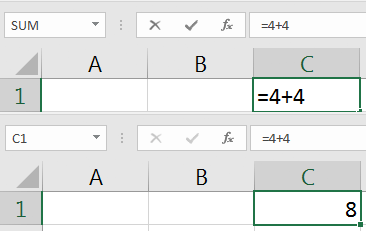Every basic Excel formula starts with the equals sign, and then the formula itself.

After the equals sign, you can put an incredible variety of things into the cell. Try typing =4+4 as your very first formula, and press enter to return the result. Excel will output 8, but the formula is still behind the scenes in the spreadsheet.

### 2. Formulas are Shown in Excel's Formula Bar

When you're typing a formula into a cell, you can see the results of the cell once you press enter. But when you select a cell, you can see the formula for that cell in the formula bar.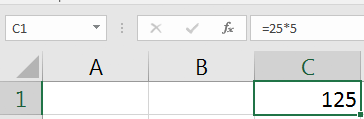Click on a cell in Excel to show the formula in it, such as the multiplication formula that evaluates to 125.

To use the example from above, the cell will show "8" , but when we click on that cell, the formula bar will show that the cell is adding 4 and 4.

### 3. How to Build a Formula

In the example above, we typed a simple addition formula to add two numbers. But, you don't have to type numbers, you can also reference other cells.

Excel is a grid of cells, with the columns running left to right, each assigned a letter, while the rows are numbered. Each cell is an intersection of a row and a column. The cell where column A and row 3 intersect is called A3, for example.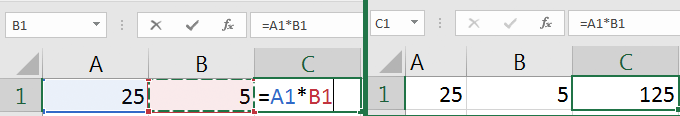Excel formulas can be written to use the values in multiple cells, such as multiplying A1 and B1 to get the value in C1, which is 125.

Let's say that I have two cells with simple numbers, like 1 and 2, and they are in cells A2 and A3. When I type a formula, I can start the formula with "=" as alwaysThen, I can type:

=A2+A3

...to add those two numbers together. It's very common to have a sheet with values, and then a separate sheet where calculations are performed. Keep all of these tips in mind while working with this tutorial. For each of the formulas, you can reference cells, or directly type numerical values into the formula.

If you need to change a formula that you've already typed, double click on the cell. You'll be able to adjust the values in the formula.

## Arithmetic in Excel

Now we'll cover Excel math formulas and walk through how to use them. Let's start off by exploring the basic arithmetic formula. These are the foundation of math operations.

### 1. Add and Subtract

Addition and subtraction are two essential math formulas in Excel. Whether you're adding up your list of business expenses for the month or balancing your checkbook digitally, the addition and subtraction operators are incredibly useful.

Use the tab titled "Add and Subtract" in the workbook for practice.

Example:

=24+48

or, reference values in cells with:

=A2+A3

Tip: try adding five or six values to see what happens. Separate each item with a plus sign.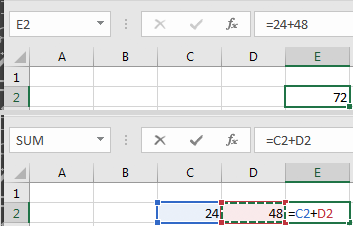Adding values in Excel is as easy as typing two numbers and a "+" sign, or adding two cell references.

#### Subtract Values

Example:

=75-25-10

or, reference values in cells with:

=A3-A2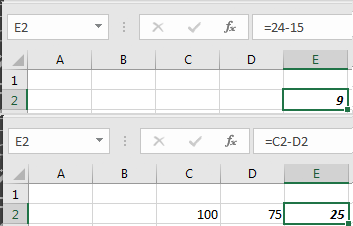Subtract two values in Excel by typing numbers directly in a box, separated with "-" sign, or reference two cells with the "-" sign between them.

### 2. Multiplication

To multiply values, use the operator. Use multiplication instead of adding the same item over and over.

Use the tab titled "Multiply" in the workbook for practice.

Example:

=5*4

or, reference values in cells with:

=A2*A3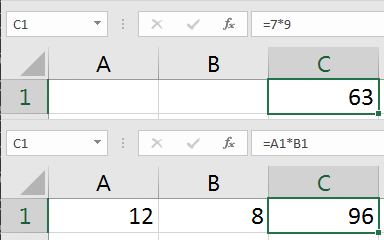Multiplication in Excel uses the * symbol between two values or cell references.

### 3. Division

Division is helpful when splitting items into groups, for example. Use the "/" operator to divide numbers or the values in cells in your spreadsheet.

Use the tab titled "Divide" in the workbook for practice.

Example:

=20/10

or, reference values in cells with:

=A5/B2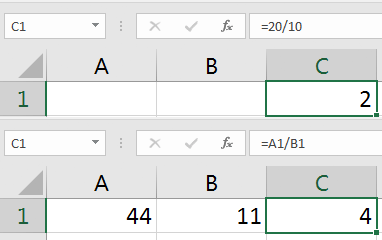Divide values in excel with the / operator, either with values in a formula or between two cells.

## Basic Statistics

Use the tab titled "Basic Statistics" in the workbook for practice.

Now that you know the basic math operators, let's move onto something more advanced. Basic statistics are helpful to review a set of data and to make informed judgments about it. Let's cover several popular, simple statistic formulas.

### 1. Average

To use an average formula in Excel, open your formula with =AVERAGE( and then input your values. Separate each number with a comma. When you press enter, Excel will calculate and output the average.

=AVERAGE(1,3,5,7,10)

The best way to calculate an average is to input your values into separate cells in a single column.

=AVERAGE(A2:A5)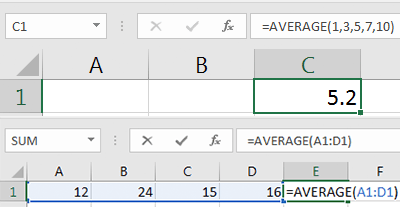Use the =AVERAGE formula to average a list of values separated by commas, or a set of cells as the bottom example shows.

### 2. Median

The median of a data set is the value that's in the middle. If you took the numerical values and ordered them from smallest to largest, the median would be smack dab in the middle of that list.

=MEDIAN(1,3,5,7,10)

I'd recommend typing your values into a list of cells, and then using the median formula over a list of cells with values typed in them.

=MEDIAN(A2:A5)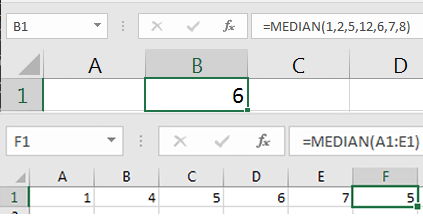Use the =MEDIAN formula to find the middle value in a list of values when you separate them with commas, or use the formula on a list of cells with values in them.

### 3. Min

If you have a set of data and want to keep your eye on the smallest value, the MIN formula in Excel is useful. You can use the MIN formula with a list of numbers, separated by commas, to find the lowest value in a set. This is very useful when working with large datasets.

=MIN(1,3,5,7,9)

You might want to find the minimum value in a list of data, which is totally possible with a formula such as:

=MIN(A1:E1)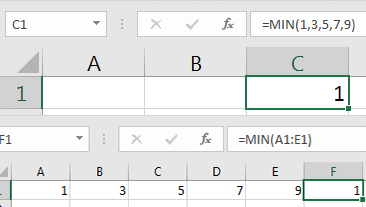Use the Excel MIN formula with a list of values separated by commas, or with a range of cells to monitor the lowest value in the set.

### 4. Max

The MAX formula in Excel is the polar opposite of MIN; it tells you which value in a set is the largest. You can use it with a list of numbers, separated by commas:

=MAX(1,3,5,7,9)

Or, you can select a list of values in cells, and have Excel return the largest in the set, with a formula like this:

=MAX(A1:E1)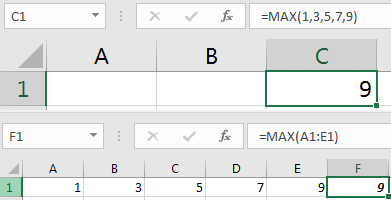The Excel MAX formula is very similar to MIN, but will help you keep an eye on the largest value in a set, and can be used on a comma separated list of values or data list.

## Recap and Keep Learning

I hope this Excel math formulas tutorial helped you think more about what Excel can do for you. With enough practice, your Excel skills will soon seem more natural than grabbing a calculator or doing math on paper. Spreadsheets capture data, and formulas help us understand or modify that data. The operations can be basic, but are at the foundation of important spreadsheets in every company.

It's always a great idea to use one tutorial to launch more learning. Here are some suggestions

What are some other math skills that you use in Excel? Leave a reply in the comments if you know a great formula that I don't.